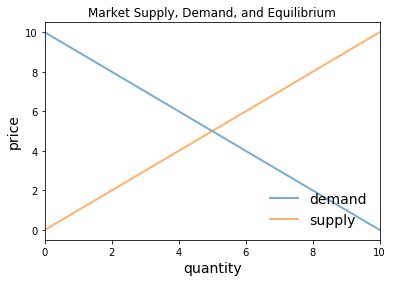# A Competitive Market ¶

Last edited: 2019-08-17

A Python class for a simple one good market where agents are price takers.

The market consists of the following objects:

A linear demand curve:

(1) $P_d = WTP_{max} - d*Q_d$

A linear supply curve:

(2) $P_s = OC_{min} + s*Q_s$

$P_d$ is the price paid by the consumer. $P_s$ is the price received by the producer. $Q_d$ is the quantity the consumer wishes to purchase at their price. $Q_s$ is the quantity the producer wishes to supply at their price. $WTP_{max}$ is the maximum willingness-to-pay of any consumer—the y-intercept of the demand curve. $OC_{min}$ is the minimum opportunity cost of any producer—the y-intercept of the supply curve. s is the slope of the supply curve. d is the slope of the demand curve.

The class provides methods to compute competitive equilibrium price and quantity, supply and demand curves, consumer surplus and producer surplus, and total surplus.

Here is the implementation:

In :
class market:

def __init__(self, WTPmax, OCmin, d, s):
"""
Set up market parameters. 𝑊𝑇𝑃𝑚𝑎𝑥 is the maximum willingness-to-pay
of any consumer—the y-intercept of the demand curve. 𝑂𝐶𝑚𝑖𝑛 is the
minimum opportunity cost of any producer—the y-intercept of the
supply curve. s is the slope of the supply curve. d is the slope
of the demand curve...
"""

self.WTPmax, self.OCmin, self.d, self.s = WTPmax, OCmin, d, s

if WTPmax < OCmin:
raise ValueError('Insufficient demand.')

def equilibrium_quantity(self):
"Compute equilibrium quantity"
return  (self.WTPmax - self.OCmin)/(self.d + self.s)

def equilibrium_price(self):
"Return equilibrium price"
return  self.WTPmax - self.d * self.equilibrium_quantity()

def consumer_surplus(self):
"Compute consumer surplus"
return (self.WTPmax - self.equilibrium_price())*self.equilibrium_quantity()/2

def producer_surplus(self):
"Compute producer surplus"
return (self.equilibrium_price() - self.OCmin) * self.equilibrium_quantity() /2

def total_surplus(self):
"Compute total surplus"
return self.producer_surplus() + self.consumer_surplus()

def demand_curve(self,x):
"Compute demand curve"
return self.WTPmax - self.d*x

def supply_curve(self,x):
"Compute supply curve"
return self.OCmin + self.s*x

In :
import numpy as np
import matplotlib.pyplot as plt
%matplotlib inline

# Baseline WTPmax, OCmin, d, s
baseline_params = 10,0,1,1
m = market(*baseline_params)

q_max = m.equilibrium_quantity() * 2
q_grid = np.linspace(0.0, q_max, 100)
pd = m.demand_curve(q_grid)
ps = m.supply_curve(q_grid)

fig, ax = plt.subplots()
ax.plot(q_grid, pd, lw=2, alpha=0.6, label='demand')
ax.plot(q_grid, ps, lw=2, alpha=0.6, label='supply')
ax.set_xlabel('quantity', fontsize=14)
ax.set_xlim(0, q_max)
ax.set_ylabel('price', fontsize=14)
ax.legend(loc='lower right', frameon=False, fontsize=14)
ax.set(title='Market Supply, Demand, and Equilibrium')
plt.show()In :
import numpy as np
import matplotlib.pyplot as plt
%matplotlib inline

# Baseline WTPmax, OCmin, d, s
baseline_params = 10,0,1,1
m = market(*baseline_params)

print(m.producer_surplus(), "= producer surplus")
print(m.consumer_surplus(), "= consumer surplus")
print(m.total_surplus(), "= total surplus")
print(m.equilibrium_quantity(), "= equilibrium quantity")
print(m.equilibrium_price(), "= equilibrium price")

12.5 = producer surplus
12.5 = consumer surplus
25.0 = total surplus
5.0 = equilibrium quantity
5.0 = equilibrium price


## A Competitive Market ¶